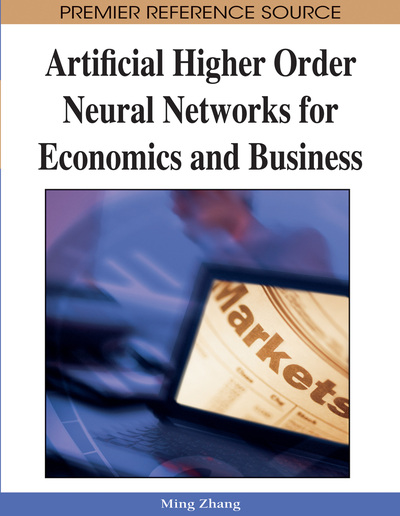# Artificial Higher Order Neural Networks in Time Series Prediction

Godfrey C. Onwubolu (University of the South Pacific, Fiji)
DOI: 10.4018/978-1-59904-897-0.ch011
Available
\$29.50
No Current Special Offers

## Abstract

Real world problems are described by nonlinear and chaotic processes, which makes them hard to model and predict. This chapter first compares the neural network (NN) and the artificial higher order neural network (HONN) and then presents commonly known neural network architectures and a number of HONN architectures. The time series prediction problem is formulated as a system identification problem, where the input to the system is the past values of a time series, and its desired output is the future values of a time series. The polynomial neural network (PNN) is then chosen as the HONN for application to the time series prediction problem. This chapter presents the application of HONN model to the nonlinear time series prediction problems of three major international currency exchange rates, as well as two key U.S. interest rates—the Federal funds rate and the yield on the 5-year U.S. Treasury note. Empirical results indicate that the proposed method is competitive with other approaches for the exchange rate problem, and can be used as a feasible solution for interest rate forecasting problem. This implies that the HONN model can be used as a feasible solution for exchange rate forecasting as well as for interest rate forecasting.
Chapter Preview
Top

## Background

### Exchange Rates Time Series

Forecasting exchange rates is an important financial problem that is receiving increasing attention especially because of its difficulty and practical applications. Exchange rates are affected by many highly correlated economic, political and even psychological factors. These factors interact in a very complex fashion. Exchange rate series exhibit high volatility, complexity and noise that result from an elusive market mechanism generating daily observations (Theodossiou, 1994).

Much research effort has been devoted to exploring the nonlinearity of exchange rate data and to developing specific nonlinear models to improve exchange rate forecasting, i.e., the autoregressive random variance (ARV) model (So et al., 1999), autoregressive conditional heteroscedasticity [ARCH] (Hsieh, 1989), self-exciting threshold autoregressive models (Chappel et al., 1996). There has been growing interest in the adoption of neural networks, fuzzy inference systems and statistical approaches for exchange rate forecasting problem (Refenes, 1993a; Refenes et al., 1993b; Yu et al., 2005a; Yu et al., 2005b). A recent review of neural networks based exchange rate forecasting is found in (Wang et al., 2004).

The input dimension (i.e. the number of delayed values for prediction) and the time delay (i.e. the time interval between two time series data) are two critical factors that affect the performance of neural networks. The selection of dimension and time delay has great significance in time series prediction.

Flexible Neural Tree [FNT] (Chen et al., 2004; Chen et al., 2005) has been used for time-series forecasting. The FNT framework, combined with an evolutionary technique, was proposed for forecasting exchange rates (Chen et al., 2006). Based on the pre-defined instruction/operator sets, a flexible neural tree model can be created and evolved. FNT allows input variables selection, over-layer connections and different activation functions for different nodes. The hierarchical structure is evolved using the Extended Compact Genetic Programming (ECGP), a tree-structure based evolutionary algorithm (Sastry and Goldberg, 2003). The fine tuning of the parameters encoded in the structure is accomplished using particle swarm optimization (PSO). In summary, they used FNT model for selecting the important inputs and/or time delays and for forecasting foreign exchange rates. Some other previous work done in predicting exchange rates include Abraham et al. 2001; Abraham et al. 2002; Onwubolu et al. 2007

## Complete Chapter List

Search this Book:
Reset